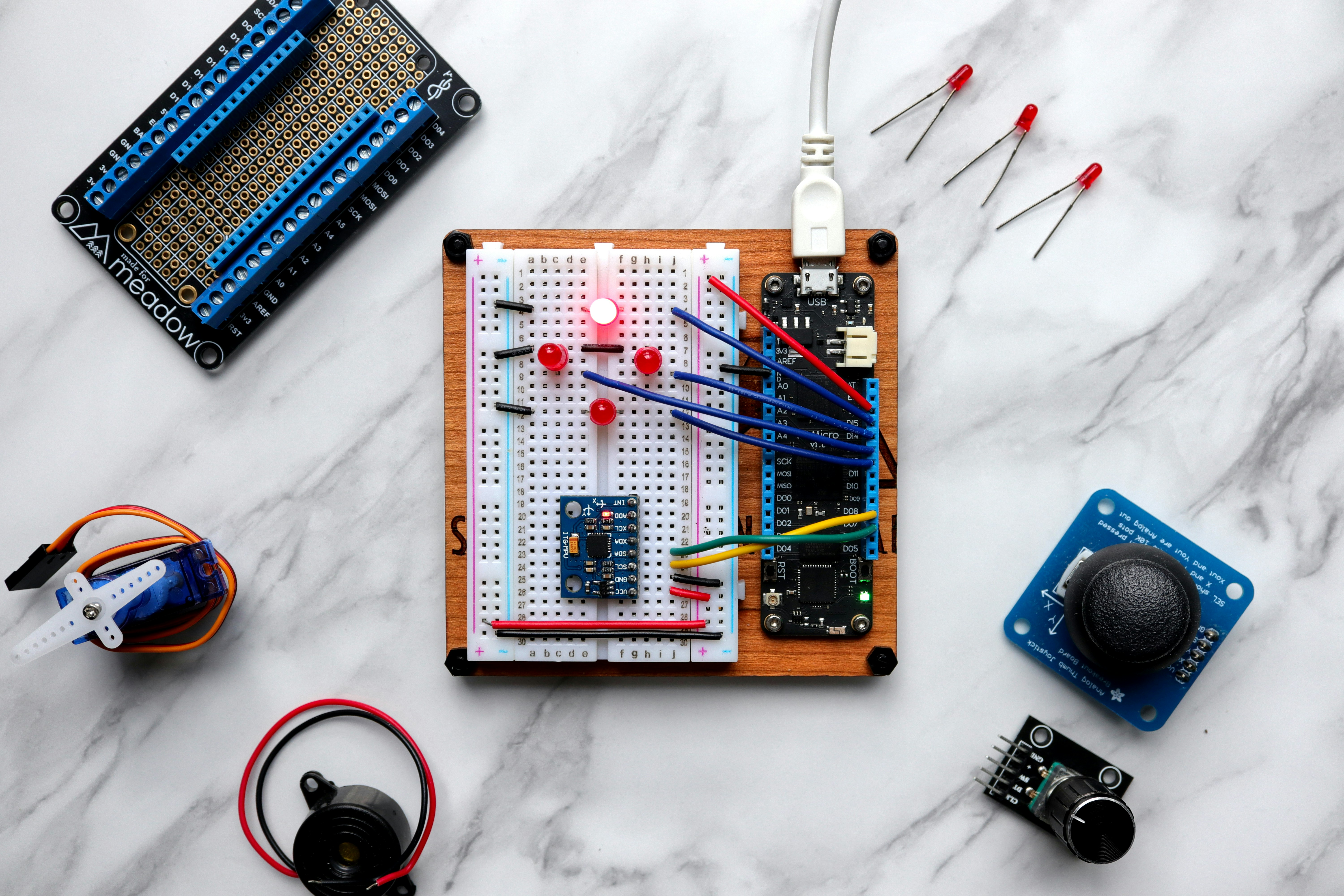Recently PublishedJournal article

## Peranan Teknologi dalam Mendukung Proses Berpikir Level C3 Siswa pada Materi Operasi Himpunan melalui Penggunaan Swish Max4Journal article

## Pengembangan LKS untuk Melatih Kemampuan Berpikir Kritis dalam Mata Pelajaran Matematika di Kelas VII SMP

Most ViewedJournal article

## Designing a Learning of Logarithm for Senior High School Students of Grade X

The study aims to know the role of logarithm learning design to introducing logarithm concept and the result at logarithm learning process through student worksheet based on Realistic Mathematics Education approach. The research method used is design research which was implemented in one of the Senior High School in Yogyakarta. The instruments used are test, video, and fieldnote. The result shows that the students look likes still adapt to learning design that implemented in the 1st and 2nd meeting, but in the next meeting the students can already start to follow learning process. Furthermore, the students result study show that the students' average value is 77.5 with frequency of completeness 65.6%. Keywords: Design Research, Logarithm Learning Designing, Realistic Mathematics EducationJournal article

## Praktikalitas Lembar Kerja Siswa pada Pembelajaran Matematika Materi Statistika

Tujuan dari penelitian ini adalah untuk mengembangkan Lembar Kerja Siswa pada pembelajaran matematika materi statistika yang praktis. Model penelitian ini menggunakan model ADDIE, yaitu tahap analisis, tahap perencanaan, tahap pengembangan, tahap implementasi, dan tahap evaluasi. Penelitian ini dibatasi pada tahap implementasi yaitu untuk melihat kepraktisan Lembar Kerja Siswa diuji coba secara terbatas. Berdasarkan hasil uji praktikalitas Lembar Kerja Siswa dengan melalui tiga data penilaian, yaitu pertama data observasi dengan rata-rata 71% kategori baik, kedua data angket respon guru dengan rata-rata 75% kategori baik, dan ketiga data angket respon siswa dengan rata-rata 84% katagori baik. &nbsp; Kata Kunci: praktikalitas, Lembar Kerja Siswa, tahap implementasi, materi statistika
Suggested For YouJournal article

## Formulasi Model Permutasi Siklis dengan Objek Multinomial

Penelitian ini bertujuan membangun model matematika untuk menghitung jumlah susunan objek dari permutasi siklis yang memiliki objek multinomial. Model yang dibangun dibatasi untuk permutasi siklis yang memiliki objek multinomial dengan minimal ada satu jenis objek beranggotakan tunggal. Pemodelan dilakukan berdasarkan struktur matematika dari permutasi siklis dan permutasi multinomial. Model permutasi siklis yang memiliki objek multinomial telah dirumuskan. &nbsp; Pembuktian model telah dilakukan melalui validasi struktur serta validasi hasil yang dilakukan dengan cara membandingkan hasil perhitungan model dan hasil pencacahan. Teorema tentang permutasi siklis dengan objek multinomial juga telah dibangun. Kata kunci:&nbsp; pemodelan , permutasi siklis, permutasi multinomial This study aims at constructing mathematical model to count the number of arrangement of objects form cyclical permutation that has multinomial objects. The model constructed is limited to cyclical permutation that has multinomial object in which at least one kind of object having single cardinality is contained within. Modelling is undertaken based on mathematical structure of cyclical permutation and multinomial permutation. Cyclical permutation model having multinomial object has been formulated as . The proof of the model has been undertaken by validating structure and validating the outcome which was conducted by comparing counting result of model and counting result manually. The theorem of cyclical permutation with multinomial object has also been developed. Keywords: modelling, cyclical permutation, multinomial permutationJournal article

## Pembelajaran Dimensi Tiga Menggunakan Pendekatan Pendidikan Matematika Realistik Indonesia (Pmri) Di SMA Negeri 2 Tanjung RajaJournal article

Journal article

## Dual Mode Error Analysis: Penyelesaian Permasalahan Luas Permukaan Serta Volume Prisma dan Limas Siswa Kelas VIII SMP